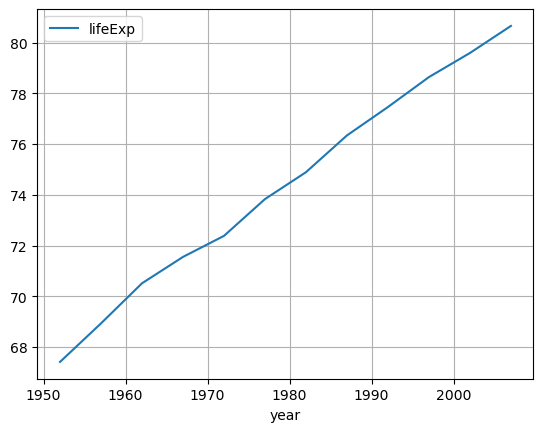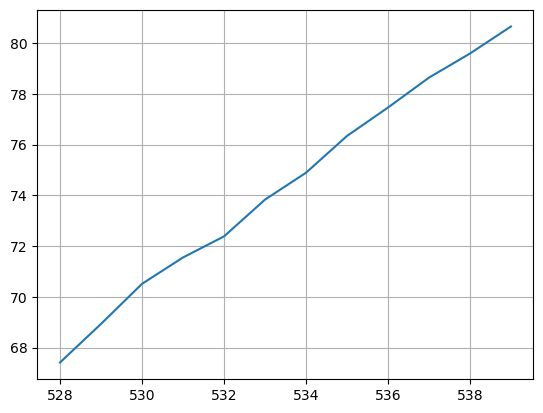## Libraries

Pandas is a popular open-source Python library used for data manipulation and analysis. It provides data structures and functions that make working with structured data, such as tabular data (like `Excel` spreadsheets or `SQL` tables), easy and intuitive.

To install Pandas, you can use the following command in your command-line interface (such as `Terminal` or `Command Prompt`):

`pip install pandas`

Matplotlib functionalities have been integrated into the pandas library, facilitating their use with `dataframes` and `series`. For this reason, you might also need to import the matplotlib library when building charts with Pandas.

This also means that they use the same functions, and if you already know Matplotlib, you'll have no trouble learning plots with Pandas.

``````import pandas as pd
import matplotlib.pyplot as plt``````

## Dataset

In order to create graphics with Pandas, we need to use pandas objects: `Dataframes` and `Series`. A dataframe can be seen as an `Excel` table, and a series as a `column` in that table. This means that we must systematically convert our data into a format used by pandas.

Since histograms need quantitative variables, we will get the Gap Minder dataset using the `read_csv()` function. The data can be accessed using the url below.

To have just one line, we create a subset of our data frame to select only the rows for France.

``````url = 'https://raw.githubusercontent.com/holtzy/The-Python-Graph-Gallery/master/static/data/gapminderData.csv'

# Subset rows for France only
df = df[df['country']=='France']``````

## Basic line chart with a Dataframe

Once we've opened our dataset, we'll now create the graph. The following displays the evolution of the life expectancy using the `plot()` function. Also, keep in mind that the `kind='line'` argument is facultative (you can remove it!) since it's the default value when calling the `plot()` function

``````# Create and display the linechart
df.plot(x='year',
y='lifeExp',
kind='line', # (facultative) Default argument
grid=True, # Add a grid in the background
)
plt.show()``````## Basic line chart with a Series

We can create the (almost) same chart using only the column of `'lifeExp'` (called a Series) of the dataframe. In this case, we do not have to specify which value will be on the `x` or `y` axis, but the x-axis will be filled with the index of the rows instead of the years.

Also, the legend will not be displayed by default (we have to put it manually if we want it)

``````# Create and display the linechart
df['lifeExp'].plot(grid=True)
plt.show()``````## Going further

This post explains how to create a simple line chart with pandas in 2 different ways (using a DataFrame and a Series).

For more examples of how to create or customize your line charts with Pandas, see the line charts section. You may also be interested in how to customize your line charts with Matplotlib and Seaborn.

## Contact & Edit

👋 This document is a work by Yan Holtz. You can contribute on github, send me a feedback on twitter or subscribe to the newsletter to know when new examples are published! 🔥